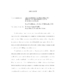## Algorithmic Approaches to Reducing Resource Costs in Data Centers2013
##### Authors
Mukherjee, Koyel
A substantial portion of resource costs incurred by data centers relate to energy costs, with cooling energy and equipment powering energy accounting for a major fraction. Other major costs incurred by data centers, is due to huge data transmission volume and resultant network bandwidth consumption. In this dissertation, we study problems inspired by the needs to reduce energy consumption and network bandwidth billing costs in data centers. A significant amount of data center cooling energy is wasted due to thermal imbalance and hot spots. In order to prevent this, the workload should be scheduled in a thermally aware manner based on the overall thermal profile, since machine temperatures depend on local load as well as on neighboring machines' load. We define effective load' that captures this spatial cross-interference and analyze different models for: 1) maximizing the profit of scheduled jobs under a cooling energy budget, for which we give 1/2 - O(epsilon) approximation algorithms; 2) minimizing the maximum temperature when all jobs have to be scheduled, for which we give a 2-approximation algorithm and a 3-competitive online algorithm for a single rack of machines, where the factors approach 4/3 and 2 respectively as the cross-interference decays. Servers consume energy while running; hence, shutting down some will reduce the costs. We consider two problems which study this in literature: active time and busy time, the goal being minimizing the total on' time of machines. In active time, we have access to a single machine whereas in busy time, number of machines allowed is unlimited. Machines have bounded capacity and jobs have release times, deadlines and lengths. For active time, we show a minimal feasible solution is 3-approximate and give a 2-approximation algorithm via LP rounding. For busy time, we give a 3-approximation algorithm which improves the 4-approximation, and analyze the preemptive problem also. Data centers need to transmit a huge volume of data daily which results in high network bandwidth costs. Frequently, ISP's charge for Internet use either based on the peak bandwidth usage in any slot in the billing cycle, or according to some percentile cost. We provide an optimal offline algorithm for the percentile problem when jobs can have variable delay. For the online problem of minimizing peak bandwidth, we study small values of delay in a discrete time setting and give much better lower and upper bounds than the best known bound of e that holds when delay allowed is arbitrarily large and time is continuous.